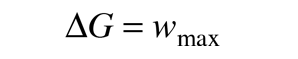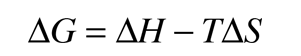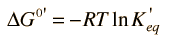# Gibbs Free Energy and the Spontaneity of Chemical Reactions

The change in Gibbs free energy associated with a chemical reaction is a useful indicator of whether the reaction will proceed spontaneously. Since the change in free energy is equal to the maximum useful work which can be accomplished by the reactionthen a negative ΔG associated with a reaction indicates that it can happen spontaneously. This is consistent with the usual chemistry convention of treating work done by the system as negative work. Most common reactions can be assessed for spontaneity under standard conditions by looking up the associated thermodynamic quantities for each of the reactants and products. For non-standard conditions one can make use of the the expression for ΔG in terms of the other thermodynamic potentialsThe change in free energy ΔG for a reaction can be expressed in terms of the equilibrium concentrations of the reactants and products. It is convenient to express ΔG for any reaction in terms of its value under standard conditions where values have been tabulated. For a reaction represented as

A + B ↔ C + D

the change in free energy can be expressed asWhere ΔG0' is the standard free energy change and it is presumed that the temperature is 25°C = 298K.

 Example of hydrolysis of ATP
Index

Electrochemistry concepts

Reference
Ebbing
Ch 18

Karp
Ch 3

 HyperPhysics***** Electricity and Magnetism ***** Chemistry R Nave
Go Back

# Standard Free Energy Change

For biochemical reactions, it is convenient to reference the change in Gibbs free energy ΔG at some standard set of conditions. The conditions usually chosen are listed in the table. The standard value ΔG0' is determined from experimental data and allows the evaluation of ΔG for other experimental conditions.

 Standard Conditions T = 25°C = 298K P = 1 atm [C]=1 M, all reactants Water 55.6M H+ conc = 10-7M (pH=7.0)The notation ΔG0' is used for pH=7.0 because ΔG0 is used for conditions pH = 0.0 or 1 M H+.

 Relation between ΔG0' and K'eq K'eq ΔG0'kcal/mol 106 -8.2 104 -5.5 102 -2.7 101 -1.4 100 0.0 10-1 1.4 10-2 2.7 10-4 5.5 10-6 8.2
Index

Electrochemistry concepts

Reference
Karp
Ch 3

 HyperPhysics***** Electricity and Magnetism ***** Chemistry R Nave
Go Back